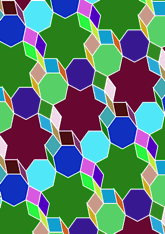# New tilings, Septagonsdata157/R7B

## Geometry

• The symmetry group of the tiling is XX (pg).
• All the internal angles of the constituent polygons are a multiple of 25.71 (π/7)°.
• Contains 6 regular two-pointed star polygons with vertex angle of 25.71 (π/7)°.
• Contains four regular two-pointed star polygons with vertex angle of 51.42 (2π/7)°.
• Contains 6 regular two-pointed star polygons with vertex angle of 77.14 (3π/7)°.
• Contains four regular heptagons.
• Contains two regular 7-pointed star polygons with vertex angle of 77.14 (3π/7)°.
• The tiling does not satisfy the two-colour condition.
• The tiling is edge-to-edge.
• As drawn, contains about 179 polygons.

## References

Publications referenced:
1. Centered tiling of Not specific. Personal Communication, Information sent to the author, 21c. [pc]

v53